﻿ Boffin Access Limited - Open Access Journals - Scholarly Publishing-STEM# ADVANCES IN COMPUTER SCIENCES (ISSN:2517-5718)

### Sacrifice Nana-Kyere1 * , Francis Agyei Boateng1, Glory Kofi Hoggar2, Paddy Jonathan3

1 Department of Mathematics, Valley View University,Kumasi campus, Ghana
2 Department of Mathematics,  Sunyani Technical University, Sunyani, Ghana
3 Department of Mathematics, Osei Kyeretwie Senior High School, Kumasi, Ghana

### CitationCitation COPIED

Kyere SN, Boateng FA, Hoggar GF, Jonathan P. Stochastic Optimal Control Model of Malaria Disease. Adv Comput Sci. 2018 Feb; 1(1):106.

### Abstract

In this research article, an optimal control model of malaria disease with standard incidence rate is proposed. Maximum Principle was employed to derive the necessary conditions for the existence of optimal control. Stochastic version of the model is derived by introducing a random perturbation in the main parameters of the model equations. Numerical solution of the optimality was derived and computed to investigate the optimum control strategy that would be efficacious to be implemented in reducing the number of exposed and infected humans as well as illustrating the explicit differences in the dynamics of the models.

### Keywords

Pontryagin’s Maximum Principle; Lagrangian; Hamiltonain; Boundary conditions; Stochastic Perturbation; Euler-Maruyama

### Introduction

The awareness that transmission dynamics of infectious diseases, the preventive and treatment strategies that are administered follow certain laws that could be modeled mathematically have been a beautiful child to the human conscience since the first incidence of epidemic outbreak .

The awakened consciousness that preventive and treatment strategies which are administered in the approach to finding solution to an epidemic outbreak require some mathematical calculations have contributed immensely in removing the subterfuge mist that has surrounded the elucidating of the transmission dynamics of infectious disease, the control strategies and the prevention of epidemic from invading a population of susceptible and has led us out into the light of strategic epidemiological control measures .

The mathematical computations which have been involved in the study of the dynamics of infectious diseases have brought a great surge of excitement in the hearts of modelers, governments, public health workers and all stakeholders as it has created awareness with regards to transmission dynamics and control measures of infectious diseases .

Concerning the malaria disease, (WHO, 2016) reported a global estimate of 212 million cases, of which the most occurred in Africa, 429000 malaria deaths, of which 303000 occurred in children under 5 years of age. These estimates rank malaria to be virulent disease and one of the top two killers among vector borne diseases. The disease is caused by parasites

that are transmitted through the bites of a host mosquito. An infected person with the disease may experience loss of appetite, headache, fever and fatigue. The disease has an adverse effect on the well-being of infected person and the task of achieving

malaria-free world throws a challenge to the public health organization which requires a strenuous and strategic efforts. The burden of the disease includes; fertility loss, premature mortality and loss of savings through treatment and medical cost, loss in worker productivity due to weakness in body. Malaria recede growth in population by means of morbidity and mortality in especially children. The disease poses threat to the development of fetal in the early stages of pregnancy in women as a result of loss of immunity. Control measures of the disease include the preventive intervention of sleeping under insecticide treated nets and spraying the breeding environments of the vector by insecticide spray and the treatment measure of seeking medical attention in the early stage of the disease .

Notwithstanding, there is an urgent need for better understanding of the disease transmission and development of effective optimal therapeutic strategies for the prevention and control of the disease due to drug and insecticide resistance challenges posed by the parasite .

Mathematical modeling has been a fruitful ground out of which have grown control therapeutic strategies for the malaria control into fruition. The contributions of mathematicamodeling to public health organizations in their intervention strategic measures of controlling malaria disease have been overwhelming . Abdullahi et al , proposed a deterministic malaria model that explains the dynamics of the disease and measures the effectiveness of anti malaria drugs in the infected compartment. Mandal et al , presented a review of existing mathematical models on malaria disease and their contributions with regards to the explanations of the transmission dynamics. Their work elaborates the evolution of modeling strategies to describe malaria incidence with the inclusion of critical features of host-vector-parasite interactions, by using an already existing mathematical model. Pariyaporn et al , used two malaria models: malaria model with standard incidence rate and malaria model with nonlinear incidence rate to explain the effect of choice of incidence function that results in occurrence of backwards bifurcation in these models. Feng et al , presented a deterministic model of malaria transmission with standard incidence rate and treatment, which was used to numerically stimulate the human data of the disease reported by the Chinese ministry of health from 2002 to 2013.

Besides, optimal controls models have come up with astonishing models coupled with various explanations of the transmission dynamics of the vector-parasite-host and have induced control therapeutic preventive strategies which could be administered for the prevention of the disease as well as treatment strategies for the treatment of the disease at a minimal cost [11-13]. Kim et al , presented a Plasmodium Vivax malaria transmission model that includes control terms by using a deterministic system of differential equations to analyze the model mathematically and numerically. Okosun et al , examined the conditions under which it is optimal to eradicate malaria disease by proposing and analyzing a deterministic model for the transmission of malaria disease that includes mass action. Fatmawati and Tasman , formulated an optimal control model of malaria transmission that considers the resistance of malaria parasite to the anti- malaria drugs. The model examined the impact of vaccination and treatment strategies on the disease transmission. Okosun et al , investigated the effectiveness and cost-effectiveness of malaria model with three preventive measures: the use of treated bednets, spraying of insecticide and a possible treatment of infective humans that blocks transmission to mosquitoes. Okosun and Makinde , examined the synergistic relationship between malaria and cholera disease in the presence of treatment by formulating a co-infection mathematical model of malaria-cholera. Mwanga et al , studied the optimal control practices of malaria. The model addresses the implementation of a catalog of optimal control strategies in the presence of uncertainties of parameter, which is generally a typical problem of infectious disease data. Rafikov et al , investigated the development of transgenic mosquitoes which may provide a new challenge and serve as an effective weapon of diseases control as a result of their resistant to diseases. Lashari et al , assessed the impact of some antimalaria control measures by reformulating an existing model as an optimal control problem. Agusto et al , applied optimal control theory to a deterministic system of differential equations to investigate an effective optimal strategy for controlling the spread of malaria disease using treatment, insecticide treated bednets and spray of mosquito insecticide as the system control variables.

Enlisted in these models are useful and successful companions and comrades in elucidating the transmission dynamics of the disease, deducing of control intervention measures to be embarked on by all stakeholders as well as insinuating imperative therapeutic strategies for medical decision making, yet there has not been a model that has been able to answer all clinical and biological information that are empirically observed regarding the disease as well as incorporating all important factors and variables in the model due to the complexity of the disease. In this research article, we consider the malaria model with standard incidence rate as proposed by , as studied in section 2. The section 3 deduces an optimal control problem that assesses the impact of some control strategies: prevention, treatment and application of insecticide spray on the vector by incorporating time dependent control functions. The necessary conditions for an optimal and the corresponding states are then derived by employing the Pontryagin’s Maximum Principle. Finally, in section 4, the resulting optimality system is numerically solved and computed to investigate the optimum control strategy that would be efficacious to be implemented in reducing the number of exposed and infected humans. Further, in section 6-7, Stochastic version of system (1) is obtained by introducing a random perturbation to the equations of the systems. In section 8, stochastic numerical method-EulerMaruyama is discussed. Section 9 gives numerical examples to show the dynamics of the deterministic, control and the stochastic systems, and to illustrate the differences in the dynamics of the models.

Model Framework

This section presents a malaria model with standard incidence rate. The human population at time t is categorized into four classes: susceptible human SH(t), exposed human EH(t), infectious human IH(t), recovered human RH(t), respectively. The mosquito population is divided into three classes: susceptible mosquito Sv (t), exposed mosquito Ev (t) and infectious mosquito Iv (t), respectively. The total populations of human and mosquito at any time are given by NH(t) = SH(t) + EH(t)+ IH(t)+ RH(t) and Nv (t)= Sv (t)+ Ev (t)+ Iv (t) respectively. The mosquito population does not include immune class as mosquitoes never recover from infection, that is, their infective period ends with their death due to their short life-cycle. Further, it is accepted that the recovered human have some level of immunity to the disease and do not get clinically ill, but still harbor low levels of parasite in their blood streams which are transmitted to a susceptible mosquitoes during bites . The mathematical differential equations of the dynamics of the malaria model as proposed by Pariyaporn et al  are:

The model assumed that people enter the susceptible class either through birth or immigration at a recruitment rate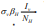. When an infectious mosquito bites a susceptible human, there is some finite probability that the malaria parasite will be passed on to the human at the rate of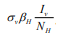, and the person will move to the exposed class. Individuals from the exposed class enter the infectious class at a rate, that is the reciprocal of the duration of the latent period. Recovered infectious humans move to the recovery class at a rate and die from the infection at a rate. It is assumed that recovered individuals have temporary immunity that can be lost and are again susceptible to re infection at a rate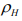. All human classes leave the population through the same natural death rate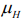. The infectious human leaves the population at a per capita disease-induced death rate. For the mosquito population, susceptible mosquitoes are recruited by birth at a constant rate, independent of the actual number of adult mosquitoes. This assumption reflects reality, since only a fraction of a large reservoir of eggs and larvae matures to the adult stage, and this process does not depend directly on the size of the adult mosquito population. All mosquito populations die due to their finite life span at the same rate of. Susceptible mosquitoes are infected by biting the infectious human and recovered human at the infection rate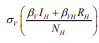Susceptible mosquitoes which are infected move to the exposed mosquito class and progress to the infectious mosquito class at a rate Vv.

Optimal Control Strategies

In this section, the state system (1) is modified to assess the impact of some control strategies: prevention, treatment and application of insecticide spray on vector. In the human population, the associated force of infection is reduced by a factor of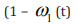where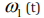represents the use of preventive control of sleeping under treated mosquito net to prevent direct contact and bite from infected mosquito.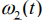represents an application of insecticide spray on the bleeding grounds of the vector. It is undeniable fact that mosquito bleeds and flourish under a favorable climate conditions such as rainy seasons and hot climatic conditions . Therefore, strenuous effort is required in application of insecticide at the bleeding fields of the vector at these times. Thus, the reproduction rate of the mosquito population is reduced by a factor of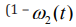) . The control function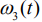measures the rate at which infected individuals are treated at each time with malaria drugs for the control of the disease. We assume that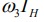individuals at any time (t) are removed from the infective class and added to the removed class. Further, we assume that the mortality rate of the vector population increases at a rate proportional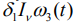, where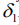> 0 is a constant rate. With regards to these underlying assumptions, an optimal control model for malaria disease is formulated that deduces prevention and treatment strategies with a minimal cost of implementation. Hence, the dynamics of system (1) are modified to the following system of equations: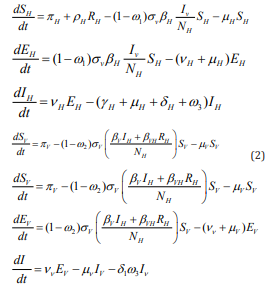The objective of our work is to minimize the number of exposed and infected human individuals and the infected vector population and maximizes the total number of recovered individuals through preventive and treatment strategies, by employing feasible minimal time dependent control variables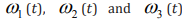respectively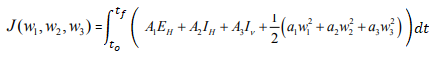(3)

With appropriate initial conditions, we consider an optimal control problem to minimize the objective functional given byThe quantities A1,A2 and A3 denote the weight constant of the exposed and infected human individuals and the infected vector population. Again, the quantities w1,w2 and w3 are weight constants for minimizing the number of exposed and infected human individuals and treatment of infected human individuals. Further, the terms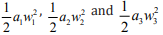represent the cost associated with the minimizing the exposed and infected and treatment of infected human individuals.

We choose a quadratic cost on the controls as a reflection of what is in other literature on epidemic control models . Here, we seek an optimal control w*1,w*2,w*3  such that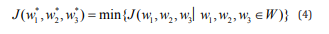Where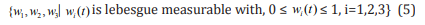Applying the Pontryagin’s Maximum Principle , the system (2) and (3) are converted into minimizing the Hamiltonian H, with respect to,  w1,w2 and w3

Where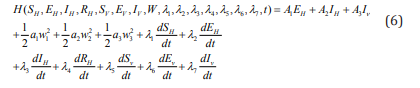Where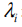for i=1,.......,7 are adjoint variables to be determined

Theorem 3.2: There exists an optimal control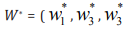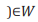such that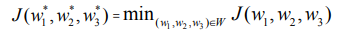subject to the control system (2) with initial conditions  at t=0.

Proof: The existence of an optimal control is proved by employing the result in . We note that the control and state variables are nonnegative values. Hence, the necessary convexity of the objective functional in w1,w2 and w3 are satisfied in this minimizing problem. The set of all control variables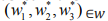is also convex and closed by definition. The optimal system is bounded which determines the compactness needed for the existence of the optimal control. In addition, the integrand in the functional (3),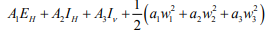is convex on the control set V. Also we can easily see that, there exist a constant V>1 and positive numbers v1,v2 such that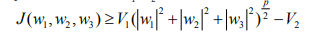because, the state variables are bounded, which completes the existence of an optimal control. In order to find the optimal solution, we apply Pontryagin’s Maximum Principle  as follows:

Given that (x,w) is an optimal solution of an optimal control problem, then there exists a non trivial vector function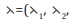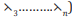which satisfies the inequalities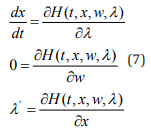Now we apply the necessary conditions to the Hamiltonian H in (6)

Theorem 3.3: Given that are optimal state solutions and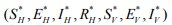are associated optimal control variable for the optimal state solutions and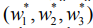are associated optimal control variable for the optimal control problem (2)-(3), then , there exists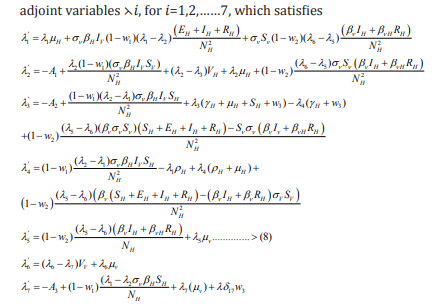with transversality conditions (or boundary conditions),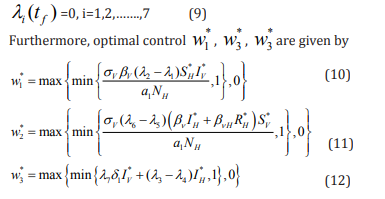Proof: The adjoint equations and the transversality conditions are determined by employing the Hamiltonian (6).By putting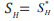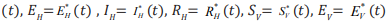and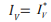differentiating the Hamiltonian with respect to SH,EH,IH,RH,SV,EV and IV respectively, we obtain (8).By solving the equation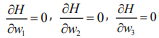on the interior of the control set and using the optimality conditions and the property of the control space W, we obtain (10)-(12).Here, we empty the formulas (10)-(12) for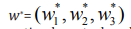the characteristic of the optimal control. The optimal control and the state are found by solving the optimality system, which consists of the state system (2), the adjoint(8), initial conditions at t=0, boundary conditions (9) , and the characterization of the optimal control (10)-(12). To solve the optimality system, we use the initial and transversality conditions together with the characterization of the optimal control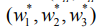given by  (10)-(12). In addition, the second derivative of the Langragian with respect to w1, w2, w3 respectively, are positive , which shows that the optimal problem is minimum at controls w*1,w*2 and w*3. By substituting the values of  w*1,w*2 and w*3.   system, we deduce the following system of equations.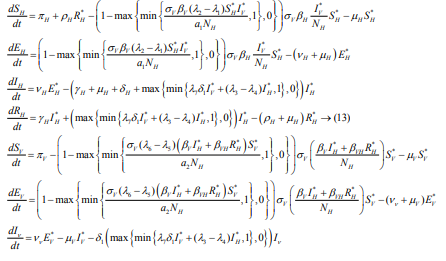With H* at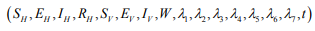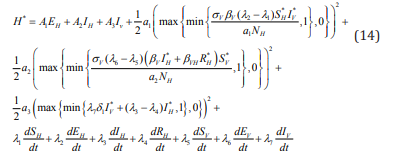Solving numerically the above systems (13) and (14) gives the optimal control and the state.

### Numerical examples and Discussion

In this section, we asses by means of numerical approach the effect of the control strategies on the transmission dynamics of malaria model. In order to achieve this, an iterative scheme is applied in solving the optimality system: state system and adjoint system. First, the state system of equations is solved with arbitrary guess for the controls over a simulated time frame by employing fourth order Runge-kutta scheme. As a result of the presence of boundary conditions, the adjoint system is solved by backwards fourth order Runge-kutta using the immediate iterative solutions of the state equation. The controls are updated by means of a convex combination of the previous controls as well as the characterizations (10), (11) and (12). The entire process is repeated until the values of the unknowns at the previous itearations are closed to the one at the current iteration.

The models assess the impact of control strategies on the transmission dynamics of Malaria disease with standard incidence rate. We investigate the effect of control strategies: prevention, treatment and application of insecticide spray on vector. This is done by numerically simulating the results of the dynamics of the disease to ascertain the stated scenarios of the strategies with parameter values  from $S_{H}\left(0\right)$=950000, $E_{H}\left(0\right)$= 500000, $I_{H}\left(0\right)$= 25000, $R_{H}\left(0\right)$=10, $S_{V}\left(0\right)$= 850000, $E_{V}\left(0\right)$= 300000, $I_{V}\left(0\right)$ = 850000, $a_{1}$= 500, $a_{2}$= 30, $a_{3}$= 10, $A_{1}$= 10, $A_{2}$= 10 and $A_{3}$= 10 from .

We further assumed that the weight factor, $a_{1}$, associated with control $w_{1}$ is greater than $A_{1}$, $A_{2}$ , $A_{3}$ , $a_{2}$ and $a_{3}$ respectively, which are association of controls $w_{2}$ and $w_{3}$. This holds on the ground that the cost of implementing $w_{1}$ includes, educational campaign programs in our public institutions such as schools, health centres and churches and media advertisements such as Television and radio educational campaigns to educate the public on the use of mosquito nets and the danger of exposing oneself to the mercy of the vector especially in pregnant women and infants. The cost of applying insecticide spray includes the insecticide cost and labour cost while treatment cost includes hospitalization, medical examination and the administration of malaria drugs in the infected persons.

The parameter values used in the simulations are estimated based on the malaria disease as provided in Table 1. Other parameters were chosen arbitrary for the purpose of the numerical simulation.

Figures 1-2 represent the number of susceptible Human ( H S ) without and with controls for a1 = 500. In the absence of control, the susceptible (solid curve) decreases gradually in the first 30 days and then rises slowly until almost the susceptible population is preserved. This is due to the fact that in the absence of control, more susceptible become infected with the disease and lose their status of being susceptible, until medical treatment has been sought. In the presence of controls, the susceptible (dashed curve) decreases sharply in the first 15 days and then experience a swift rise in the population, until almost their population are maintained in about 100days of the infection. This is possible due to the negligence, errors and difficulty of implementing these control measures, thereby exposing more susceptible to the vector, causing a lot of casualties in the early stages of the pandemic. But when these measures are properly instituted and monitored, the situation is reversed, thereby experiencing a swift rise in the curve.

Similarly, figures 3-4 represent the number of Susceptible Mosquito ( V S ) without and with controls for a1 = 500. When there are no controls, the Susceptible Mosquito (solid curve) decreases sharply in the first 50 days until all the susceptible population are infected with the disease and leaves no population of susceptible. This is due to the fact that in the absence of any effective control measure, the mosquito-human contact is not prevented and the vector gets contact with human easily and feeds on his blood, thereby causing more people to be infected with the disease. However, in the presence of controls, the mosquito-human contact is minimized to some extent causing fewer people to be infected with the disease. Hence, there is a gradual decrease in the control susceptible mosquito curve (dashed curve) for the first 70 days until all the population gets infected with the disease.

Figures 5-6 represent the number of Exposed Human ( EH ) without and with controls for a1 = 500. Evidently, in the absence of control, the mosquito-human contact is not prevented. Hence, more people are expected to be exposed to the disease. This is shown in the sudden rise of the exposed curve without control (solid curve) in the early stages of 20 days and rises until almost all the population becomes exposed to the malaria disease. In the presence of controls, the mosquito-human contact is minimized drastically and people exposed to the disease eventually gain immunity through treatment or stronger immune system. Hence, the curve with control (dashed curve) experiences a gradual decrease for the first 60 days and decreases until all the exposed human leaves the population.

Further, Figures 7-8 represent the number of Exposed Mosquito ( EV ) without and with controls for a1 = 500. In the absence of control, the exposed mosquito (solid curve) decreases gradually for the first 50 days until all the exposed population is infected and leaves no population of exposed. In the presence of controls, the exposed mosquito (dashed curve) decreases sharply, and their population are maintained until about 30 days where all their population degenerated due to being infected. This is due to the fact that in the presence of controls, the mosquito- human contact is minimized and this prevent more mosquitoes from getting exposed, and enhance the exposed mosquitoes from leaving the population at a faster rate.

Figures 9-10 represent the number of Infected Human ( HI ) without and with controls for a1 = 500. It can be ascertained that, in the absence of controls, the mosquito-human contact is not prevented. More humans would be infected when they are bitten by mosquitoes. This is reflected in the infected human curve (solid curve). In the absence of controls, the curve rises sharply in the first 10 days and rises until it maintains its level for the rest of the days, until almost all the population becomes infected. The presence of controls is witnessed by an unprecedented rise in the infected human curve (dashed curve) which however reverse within 5 days and decreases sharply for the next 55 days where the infected human degenerates and leaves no population of infected. This suggests that the control strategies proposed were effective in minimizing the infected human population drastically.

Similarly, figures 11-12 represent the number of Infected Mosquito ( VI ) without and with controls for a1 = 500. In the absence of controls, the infected mosquito curve (solid curve) decreases slowly and the infected mosquitoes are not completely removed from the population at the end of 100 days.

However, with the introduction of controls, the infected mosquito curve (dashed curve) decreases sharply and the infected mosquitoes are wiped out from the population before 70 days. This insinuates that the control strategies proposed have been effective in minimizing the infected mosquito population drastically.

Figures 13-15, present an optimal control effort to educate the public of the use and importance of implementing the preventive control of sleeping under treated mosquito net to prevent direct contact and bite from infected mosquito, w1 , the application of insecticide spray on the bleeding grounds of the vector, w2 and the rate at which infected human seeks medical treatment, w3 . The plots indicates that the preventive control w1 was at the upper bound for = 72, before it decreases gradually to the lower bound at = 100, while the optimal preventive control w2 and treatment control 3 both maintain the maximum of 100% for = 99 before they drop sharply to the lower bound at = 100. This implies that minimum effort would be required for implementing the application of the insecticide spray on the bleeding grounds of the vector and treatment of the infected human than implementation of the preventive control of sleeping under mosquito net at a1 = 10000.

Similarly, figures 16-18 represent the control effort of implementing the preventive control of sleeping under treated mosquito net, w1 , the application of insecticide spray, w2 and the rate at which infected human seeks medical treatment, w3 . Here, we observed that the preventive control w1 was at the upper bound for = 98, dropping gradually to the lower bound at = 100, while the optimal preventive control w2 and treatment control 3 both maintain the maximum of 100% for = 99 before they drop sharply to the lower bound at = 100.

This suggests that minimal effort is required to implement the application of the insecticide spray on the bleeding grounds of the vector and treatment of the infected human than implementation of the preventive control of sleeping under mosquito net at a2 = 10000.

Finally, figures 19-21 represent the control effort of implementing the preventive control of sleeping under treated mosquito net, w1 , the application of insecticide spray, w2 and the rate at which infected human seeks medical treatment, w3 . Again, we see that the preventive control w1 was at the upper bound for = 98, dropping gradually to the lower bound at = 100, while the optimal preventive control w2 and treatment control w3 both maintain the maximum of 100% for = 99 before they drop sharply to the lower bound at = 100. Hence, this suggests that minimal effort is required to implement the application of the insecticide spray on the bleeding grounds of the vector and treatment of the infected human than implementation of the preventive control of sleeping under mosquito net at a3 = 10000.

In this research article, an optimal control model of malaria disease with standard incidence rate was mathematically formulated to study the transmission dynamics of the disease in order to analyze the optimum control strategy that would be efficacious to be implemented to control the disease at a minimal cost. Three control functions were introduced to assess and measure empirically the efficacy of the use of sleeping under treated mosquito net to prevent direct contact and bite from infected mosquito, the application of insecticide spray on the bleeding grounds of the vector and the rate at which infected humans are treated at each time of the infection. The analysis proved that the optimal control strategies considered have an optimum and incomparable results on the reduction of the number of exposed and infected humans and mosquitoes as compared to the model without control as illustrated in the plot of figures for the models with and without controls. The numerical examples depict that despite the standard incidence rate, the proposed strategies are effective in the reduction of the number of the exposed and infected human and mosquito of the disease.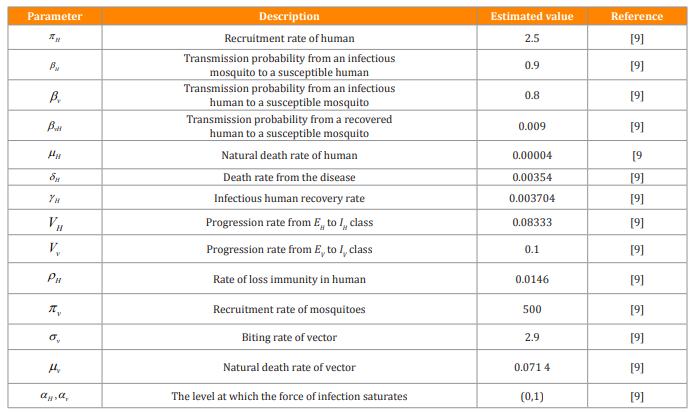Table 1: Description of variables and parameters of the Malaria Model (1)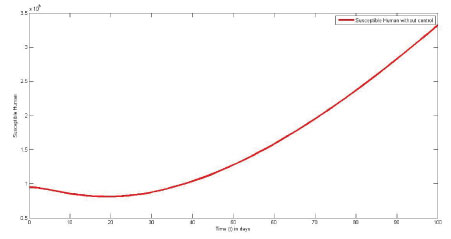Figure 1: The plot represents population of susceptible Human without control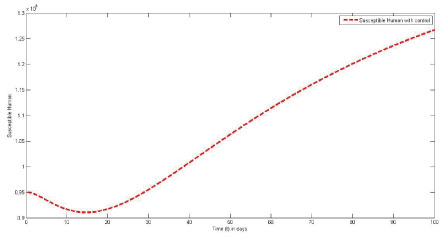Figure 2: The plot represents population of susceptible Human with control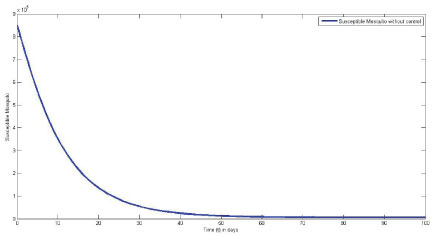Figure 3: The plot represents population of susceptible Mosquito without control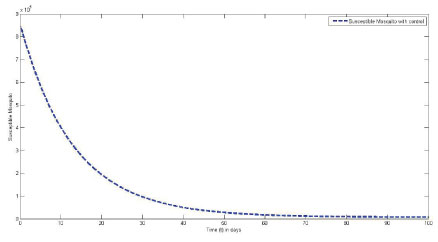Figure 4: The plot represents population of susceptible Mosquito with control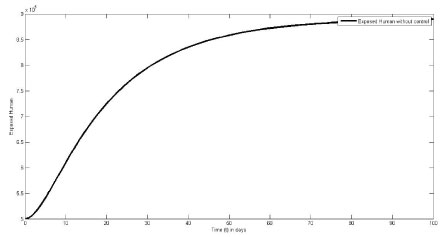Figure 5: The plot represents population of Exposed Human without control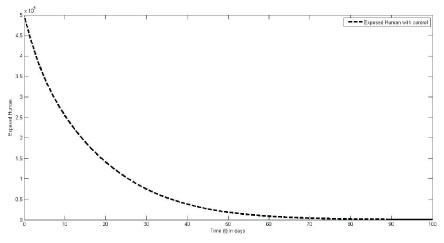Figure 6: The plot represents population of Exposed Human with control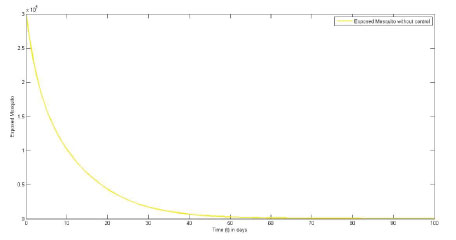Figure 7: The plot represents population of Exposed Mosquito without control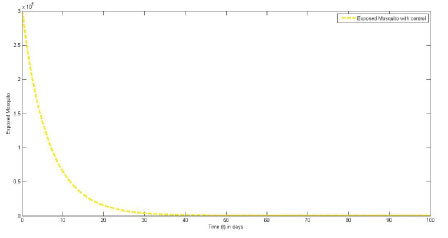Figure 8: The plot represents population of Exposed Mosquito with control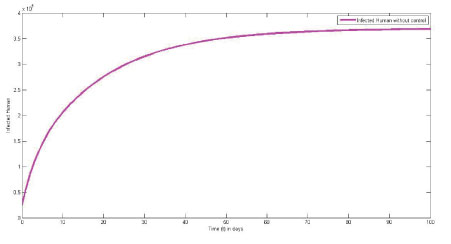Figure 9: The plot represents population of Infected Human without control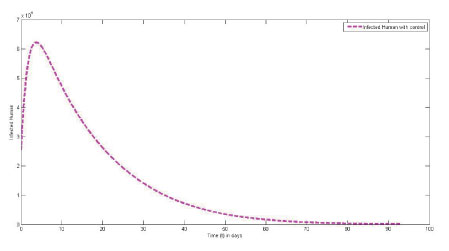Figure 10: The plot represents population of Infected Human with control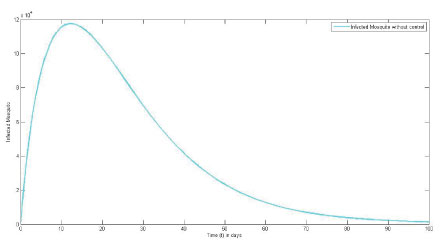Figure 11: The plot represents population of Infected Mosquito without control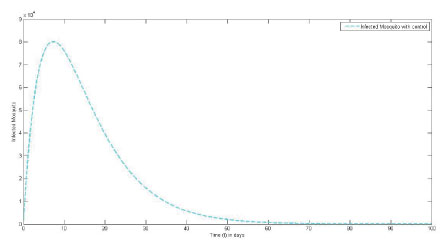Figure 12: The plot represents population of Infected Mosquito with control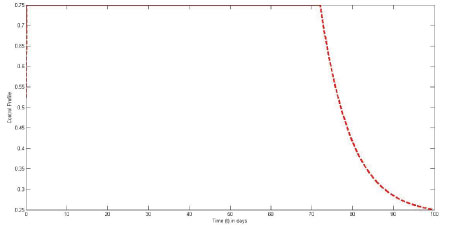Figure 13: The plot represents Optimal control $w_{1}$  with $a_{1}$=10000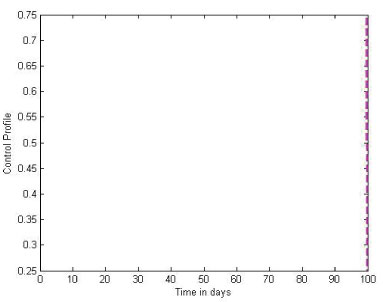Figure 14: The plot represents Optimal control $w_{2}$ with $a_{1}$=10000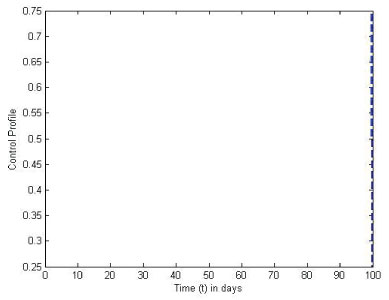Figure 15: The plot represents Optimal control $w_{3}$ with $a_{1}$=10000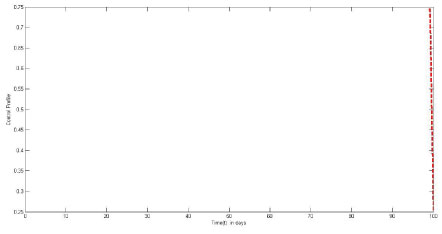Figure 16: The plot represents Optimal control $w_{1}$ with $a_{2}$=10000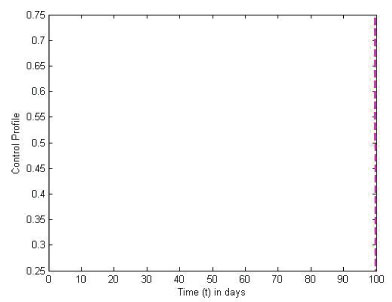Figure 17: The plot represents Optimal control $w_{2}$ with $a_{2}$=10000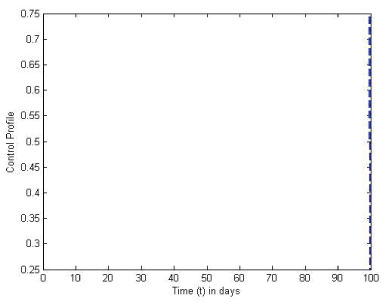Figure 18: The plot represents Optimal control $w_{3}$ with $a_{2}$=10000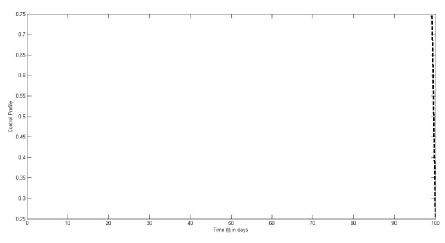Figure 19: The plot represents Optimal control $w_{1}$ with $a_{3}$=10000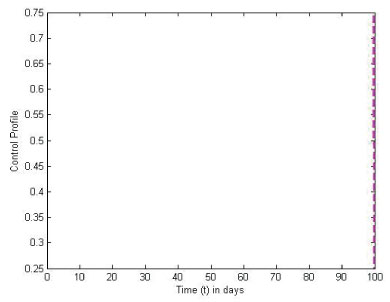Figure 20: The plot represents Optimal control $w_{2}$ with $a_{1}$=10000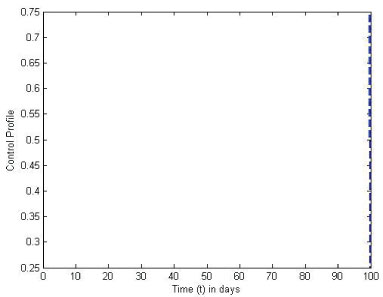Figure 21: The plot represents Optimal control $w_{3}$with $a_{3}$=10000

### Conclusion

In this research article, an optimal control model of malaria disease with standard incidence rate was mathematically formulated to study the transmission dynamics of the disease in order to analyze the optimum control strategy that would be efficacious to be implemented to control the disease at a minimal cost. Three control functions were introduced to assess and measure empirically the efficacy of the use of sleeping under treated mosquito net to prevent direct contact and bite from infected mosquito, the application of insecticide spray on the bleeding grounds of the vector and the rate at which infected humans are treated at each time of the infection. The analysis proved that the optimal control strategies considered have an optimum and incomparable results on the reduction of the number of exposed and infected humans and mosquitoes as compared to the model without control as illustrated in the plot of figures for the models with and without controls. The numerical examples depict that despite the standard incidence rate, the proposed strategies are effective in the reduction of the number of the exposed and infected human and mosquito of the disease.